GFG App
Open AppBrowser
Continue

# Kruskal’s Minimum Spanning Tree (MST) Algorithm

A minimum spanning tree (MST) or minimum weight spanning tree for a weighted, connected, undirected graph is a spanning tree with a weight less than or equal to the weight of every other spanning tree. To learn more about Minimum Spanning Tree, refer to this article.

## Introduction to Kruskal’s Algorithm:

Here we will discuss Kruskal’s algorithm to find the MST of a given weighted graph.

In Kruskal’s algorithm, sort all edges of the given graph in increasing order. Then it keeps on adding new edges and nodes in the MST if the newly added edge does not form a cycle. It picks the minimum weighted edge at first at the maximum weighted edge at last. Thus we can say that it makes a locally optimal choice in each step in order to find the optimal solution. Hence this is a Greedy Algorithm.

## How to find MST using Kruskal’s algorithm?

Below are the steps for finding MST using Kruskal’s algorithm:

1. Sort all the edges in non-decreasing order of their weight.
2. Pick the smallest edge. Check if it forms a cycle with the spanning tree formed so far. If the cycle is not formed, include this edge. Else, discard it.
3. Repeat step#2 until there are (V-1) edges in the spanning tree.

Step 2 uses the Union-Find algorithm to detect cycles.

So we recommend reading the following post as a prerequisite.

Kruskal’s algorithm to find the minimum cost spanning tree uses the greedy approach. The Greedy Choice is to pick the smallest weight edge that does not cause a cycle in the MST constructed so far. Let us understand it with an example:

### Illustration:

Below is the illustration of the above approach:

Input Graph: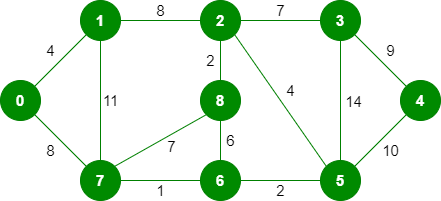The graph contains 9 vertices and 14 edges. So, the minimum spanning tree formed will be having (9 – 1) = 8 edges.
After sorting:

Now pick all edges one by one from the sorted list of edges

Step 1: Pick edge 7-6. No cycle is formed, include it.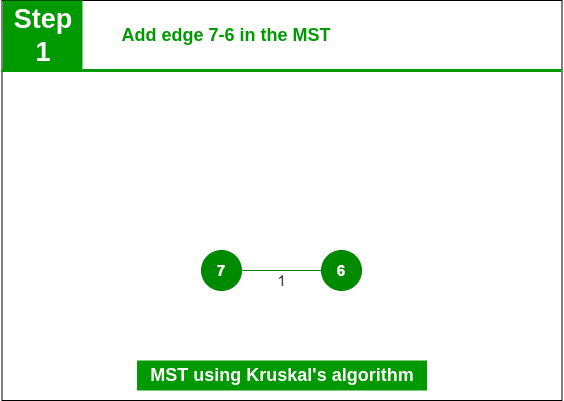Add edge 7-6 in the MST

Step 2:  Pick edge 8-2. No cycle is formed, include it.Add edge 8-2 in the MST

Step 3: Pick edge 6-5. No cycle is formed, include it.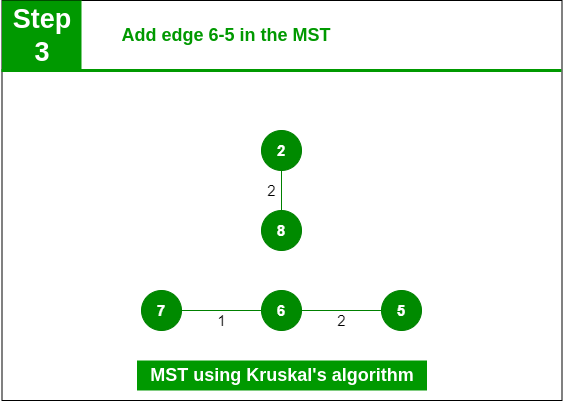Add edge 6-5 in the MST

Step 4: Pick edge 0-1. No cycle is formed, include it.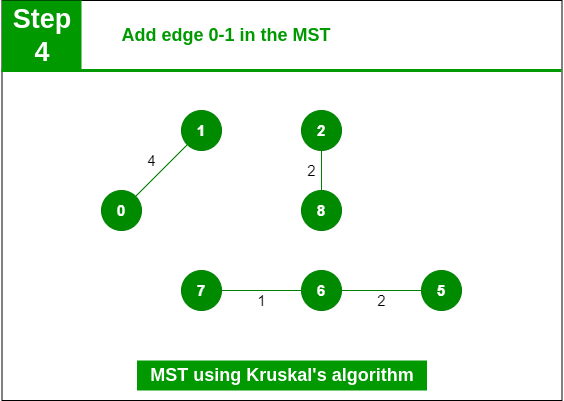Add edge 0-1 in the MST

Step 5: Pick edge 2-5. No cycle is formed, include it.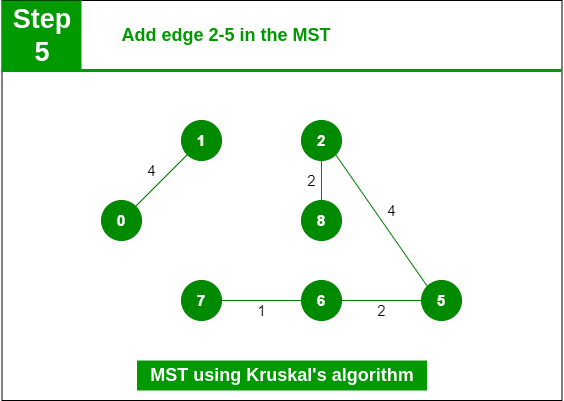Add edge 2-5 in the MST

Step 6: Pick edge 8-6. Since including this edge results in the cycle, discard it. Pick edge 2-3: No cycle is formed, include it.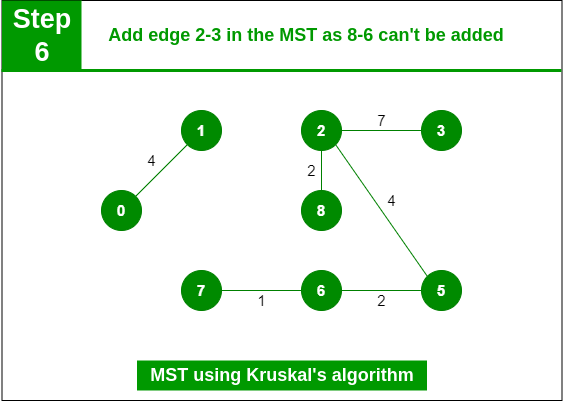Add edge 2-3 in the MST

Step 7: Pick edge 7-8. Since including this edge results in the cycle, discard it. Pick edge 0-7. No cycle is formed, include it.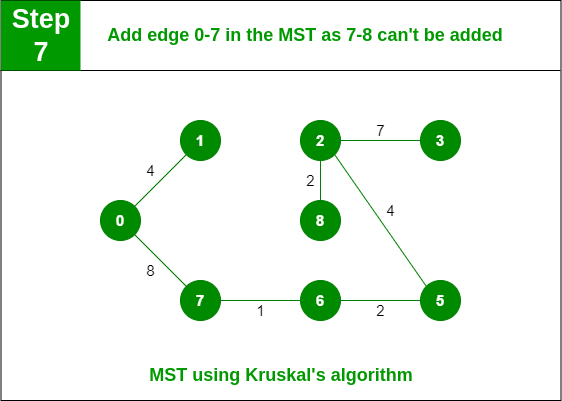Step 8: Pick edge 1-2. Since including this edge results in the cycle, discard it. Pick edge 3-4. No cycle is formed, include it.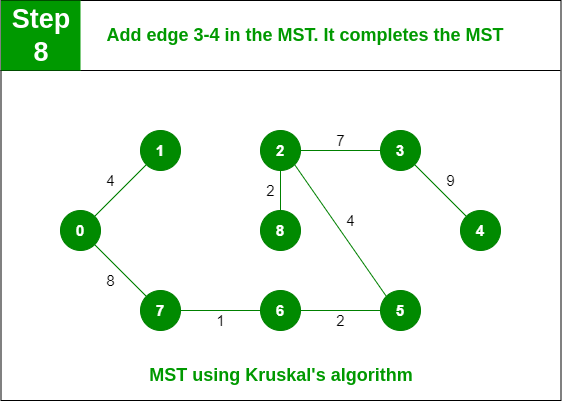Add edge 3-4 in the MST

Note: Since the number of edges included in the MST equals to (V – 1), so the algorithm stops here

Below is the implementation of the above approach:

## C

 `// C code to implement Kruskal's algorithm`   `#include ` `#include `   `// Comparator function to use in sorting` `int` `comparator(``const` `void``* p1, ``const` `void``* p2)` `{` `    ``const` `int``(*x) = p1;` `    ``const` `int``(*y) = p2;`   `    ``return` `(*x) - (*y);` `}`   `// Initialization of parent[] and rank[] arrays` `void` `makeSet(``int` `parent[], ``int` `rank[], ``int` `n)` `{` `    ``for` `(``int` `i = 0; i < n; i++) {` `        ``parent[i] = i;` `        ``rank[i] = 0;` `    ``}` `}`   `// Function to find the parent of a node` `int` `findParent(``int` `parent[], ``int` `component)` `{` `    ``if` `(parent[component] == component)` `        ``return` `component;`   `    ``return` `parent[component]` `           ``= findParent(parent, parent[component]);` `}`   `// Function to unite two sets` `void` `unionSet(``int` `u, ``int` `v, ``int` `parent[], ``int` `rank[], ``int` `n)` `{` `    ``// Finding the parents` `    ``u = findParent(parent, u);` `    ``v = findParent(parent, v);`   `    ``if` `(rank[u] < rank[v]) {` `        ``parent[u] = v;` `    ``}` `    ``else` `if` `(rank[u] > rank[v]) {` `        ``parent[v] = u;` `    ``}` `    ``else` `{` `        ``parent[v] = u;`   `        ``// Since the rank increases if` `        ``// the ranks of two sets are same` `        ``rank[u]++;` `    ``}` `}`   `// Function to find the MST` `void` `kruskalAlgo(``int` `n, ``int` `edge[n])` `{` `    ``// First we sort the edge array in ascending order` `    ``// so that we can access minimum distances/cost` `    ``qsort``(edge, n, ``sizeof``(edge), comparator);`   `    ``int` `parent[n];` `    ``int` `rank[n];`   `    ``// Function to initialize parent[] and rank[]` `    ``makeSet(parent, rank, n);`   `    ``// To store the minimun cost` `    ``int` `minCost = 0;`   `    ``printf``(` `        ``"Following are the edges in the constructed MST\n"``);` `    ``for` `(``int` `i = 0; i < n; i++) {` `        ``int` `v1 = findParent(parent, edge[i]);` `        ``int` `v2 = findParent(parent, edge[i]);` `        ``int` `wt = edge[i];`   `        ``// If the parents are different that` `        ``// means they are in different sets so` `        ``// union them` `        ``if` `(v1 != v2) {` `            ``unionSet(v1, v2, parent, rank, n);` `            ``minCost += wt;` `            ``printf``(``"%d -- %d == %d\n"``, edge[i],` `                   ``edge[i], wt);` `        ``}` `    ``}`   `    ``printf``(``"Minimum Cost Spanning Tree: %d\n"``, minCost);` `}`   `// Driver code` `int` `main()` `{` `    ``int` `edge = { { 0, 1, 10 },` `                       ``{ 0, 2, 6 },` `                       ``{ 0, 3, 5 },` `                       ``{ 1, 3, 15 },` `                       ``{ 2, 3, 4 } };`   `    ``kruskalAlgo(5, edge);`   `    ``return` `0;` `}`

## C++

 `// C++ program for the above approach`   `#include ` `using` `namespace` `std;`   `// DSU data structure` `// path compression + rank by union` `class` `DSU {` `    ``int``* parent;` `    ``int``* rank;`   `public``:` `    ``DSU(``int` `n)` `    ``{` `        ``parent = ``new` `int``[n];` `        ``rank = ``new` `int``[n];`   `        ``for` `(``int` `i = 0; i < n; i++) {` `            ``parent[i] = -1;` `            ``rank[i] = 1;` `        ``}` `    ``}`   `    ``// Find function` `    ``int` `find(``int` `i)` `    ``{` `        ``if` `(parent[i] == -1)` `            ``return` `i;`   `        ``return` `parent[i] = find(parent[i]);` `    ``}`   `    ``// Union function` `    ``void` `unite(``int` `x, ``int` `y)` `    ``{` `        ``int` `s1 = find(x);` `        ``int` `s2 = find(y);`   `        ``if` `(s1 != s2) {` `            ``if` `(rank[s1] < rank[s2]) {` `                ``parent[s1] = s2;` `            ``}` `            ``else` `if` `(rank[s1] > rank[s2]) {` `                ``parent[s2] = s1;` `            ``}` `            ``else` `{` `                ``parent[s2] = s1;` `                ``rank[s1] += 1;` `            ``}` `        ``}` `    ``}` `};`   `class` `Graph {` `    ``vector > edgelist;` `    ``int` `V;`   `public``:` `    ``Graph(``int` `V) { ``this``->V = V; }`   `    ``// Function to add edge in a graph` `    ``void` `addEdge(``int` `x, ``int` `y, ``int` `w)` `    ``{` `        ``edgelist.push_back({ w, x, y });` `    ``}`   `    ``void` `kruskals_mst()` `    ``{` `        ``// Sort all edges` `        ``sort(edgelist.begin(), edgelist.end());`   `        ``// Initialize the DSU` `        ``DSU s(V);` `        ``int` `ans = 0;` `        ``cout << ``"Following are the edges in the "` `                ``"constructed MST"` `             ``<< endl;` `        ``for` `(``auto` `edge : edgelist) {` `            ``int` `w = edge;` `            ``int` `x = edge;` `            ``int` `y = edge;`   `            ``// Take this edge in MST if it does` `            ``// not forms a cycle` `            ``if` `(s.find(x) != s.find(y)) {` `                ``s.unite(x, y);` `                ``ans += w;` `                ``cout << x << ``" -- "` `<< y << ``" == "` `<< w` `                     ``<< endl;` `            ``}` `        ``}` `        ``cout << ``"Minimum Cost Spanning Tree: "` `<< ans;` `    ``}` `};`   `// Driver code` `int` `main()` `{` `    ``Graph g(4);` `    ``g.addEdge(0, 1, 10);` `    ``g.addEdge(1, 3, 15);` `    ``g.addEdge(2, 3, 4);` `    ``g.addEdge(2, 0, 6);` `    ``g.addEdge(0, 3, 5);`   `    ``// Function call` `    ``g.kruskals_mst();`   `    ``return` `0;` `}`

## Java

 `// Java program for Kruskal's algorithm`   `import` `java.util.ArrayList;` `import` `java.util.Comparator;` `import` `java.util.List;`   `public` `class` `KruskalsMST {`   `    ``// Defines edge structure` `    ``static` `class` `Edge {` `        ``int` `src, dest, weight;`   `        ``public` `Edge(``int` `src, ``int` `dest, ``int` `weight)` `        ``{` `            ``this``.src = src;` `            ``this``.dest = dest;` `            ``this``.weight = weight;` `        ``}` `    ``}`   `    ``// Defines subset element structure` `    ``static` `class` `Subset {` `        ``int` `parent, rank;`   `        ``public` `Subset(``int` `parent, ``int` `rank)` `        ``{` `            ``this``.parent = parent;` `            ``this``.rank = rank;` `        ``}` `    ``}`   `    ``// Starting point of program execution` `    ``public` `static` `void` `main(String[] args)` `    ``{` `        ``int` `V = ``4``;` `        ``List graphEdges = ``new` `ArrayList(` `            ``List.of(``new` `Edge(``0``, ``1``, ``10``), ``new` `Edge(``0``, ``2``, ``6``),` `                    ``new` `Edge(``0``, ``3``, ``5``), ``new` `Edge(``1``, ``3``, ``15``),` `                    ``new` `Edge(``2``, ``3``, ``4``)));`   `        ``// Sort the edges in non-decreasing order` `        ``// (increasing with repetition allowed)` `        ``graphEdges.sort(``new` `Comparator() {` `            ``@Override` `public` `int` `compare(Edge o1, Edge o2)` `            ``{` `                ``return` `o1.weight - o2.weight;` `            ``}` `        ``});`   `        ``kruskals(V, graphEdges);` `    ``}`   `    ``// Function to find the MST` `    ``private` `static` `void` `kruskals(``int` `V, List edges)` `    ``{` `        ``int` `j = ``0``;` `        ``int` `noOfEdges = ``0``;`   `        ``// Allocate memory for creating V subsets` `        ``Subset subsets[] = ``new` `Subset[V];`   `        ``// Allocate memory for results` `        ``Edge results[] = ``new` `Edge[V];`   `        ``// Create V subsets with single elements` `        ``for` `(``int` `i = ``0``; i < V; i++) {` `            ``subsets[i] = ``new` `Subset(i, ``0``);` `        ``}`   `        ``// Number of edges to be taken is equal to V-1` `        ``while` `(noOfEdges < V - ``1``) {`   `            ``// Pick the smallest edge. And increment` `            ``// the index for next iteration` `            ``Edge nextEdge = edges.get(j);` `            ``int` `x = findRoot(subsets, nextEdge.src);` `            ``int` `y = findRoot(subsets, nextEdge.dest);`   `            ``// If including this edge doesn't cause cycle,` `            ``// include it in result and increment the index` `            ``// of result for next edge` `            ``if` `(x != y) {` `                ``results[noOfEdges] = nextEdge;` `                ``union(subsets, x, y);` `                ``noOfEdges++;` `            ``}`   `            ``j++;` `        ``}`   `        ``// Print the contents of result[] to display the` `        ``// built MST` `        ``System.out.println(` `            ``"Following are the edges of the constructed MST:"``);` `        ``int` `minCost = ``0``;` `        ``for` `(``int` `i = ``0``; i < noOfEdges; i++) {` `            ``System.out.println(results[i].src + ``" -- "` `                               ``+ results[i].dest + ``" == "` `                               ``+ results[i].weight);` `            ``minCost += results[i].weight;` `        ``}` `        ``System.out.println(``"Total cost of MST: "` `+ minCost);` `    ``}`   `    ``// Function to unite two disjoint sets` `    ``private` `static` `void` `union(Subset[] subsets, ``int` `x,` `                              ``int` `y)` `    ``{` `        ``int` `rootX = findRoot(subsets, x);` `        ``int` `rootY = findRoot(subsets, y);`   `        ``if` `(subsets[rootY].rank < subsets[rootX].rank) {` `            ``subsets[rootY].parent = rootX;` `        ``}` `        ``else` `if` `(subsets[rootX].rank` `                 ``< subsets[rootY].rank) {` `            ``subsets[rootX].parent = rootY;` `        ``}` `        ``else` `{` `            ``subsets[rootY].parent = rootX;` `            ``subsets[rootX].rank++;` `        ``}` `    ``}`   `    ``// Function to find parent of a set` `    ``private` `static` `int` `findRoot(Subset[] subsets, ``int` `i)` `    ``{` `        ``if` `(subsets[i].parent == i)` `            ``return` `subsets[i].parent;`   `        ``subsets[i].parent` `            ``= findRoot(subsets, subsets[i].parent);` `        ``return` `subsets[i].parent;` `    ``}` `}` `// This code is contributed by Salvino D'sa`

## Python3

 `# Python program for Kruskal's algorithm to find` `# Minimum Spanning Tree of a given connected,` `# undirected and weighted graph`     `# Class to represent a graph` `class` `Graph:`   `    ``def` `__init__(``self``, vertices):` `        ``self``.V ``=` `vertices` `        ``self``.graph ``=` `[]`   `    ``# Function to add an edge to graph` `    ``def` `addEdge(``self``, u, v, w):` `        ``self``.graph.append([u, v, w])`   `    ``# A utility function to find set of an element i` `    ``# (truly uses path compression technique)` `    ``def` `find(``self``, parent, i):` `        ``if` `parent[i] !``=` `i:`   `            ``# Reassignment of node's parent` `            ``# to root node as` `            ``# path compression requires` `            ``parent[i] ``=` `self``.find(parent, parent[i])` `        ``return` `parent[i]`   `    ``# A function that does union of two sets of x and y` `    ``# (uses union by rank)` `    ``def` `union(``self``, parent, rank, x, y):`   `        ``# Attach smaller rank tree under root of` `        ``# high rank tree (Union by Rank)` `        ``if` `rank[x] < rank[y]:` `            ``parent[x] ``=` `y` `        ``elif` `rank[x] > rank[y]:` `            ``parent[y] ``=` `x`   `        ``# If ranks are same, then make one as root` `        ``# and increment its rank by one` `        ``else``:` `            ``parent[y] ``=` `x` `            ``rank[x] ``+``=` `1`   `    ``# The main function to construct MST` `    ``# using Kruskal's algorithm` `    ``def` `KruskalMST(``self``):`   `        ``# This will store the resultant MST` `        ``result ``=` `[]`   `        ``# An index variable, used for sorted edges` `        ``i ``=` `0`   `        ``# An index variable, used for result[]` `        ``e ``=` `0`   `        ``# Sort all the edges in` `        ``# non-decreasing order of their` `        ``# weight` `        ``self``.graph ``=` `sorted``(``self``.graph,` `                            ``key``=``lambda` `item: item[``2``])`   `        ``parent ``=` `[]` `        ``rank ``=` `[]`   `        ``# Create V subsets with single elements` `        ``for` `node ``in` `range``(``self``.V):` `            ``parent.append(node)` `            ``rank.append(``0``)`   `        ``# Number of edges to be taken is less than to V-1` `        ``while` `e < ``self``.V ``-` `1``:`   `            ``# Pick the smallest edge and increment` `            ``# the index for next iteration` `            ``u, v, w ``=` `self``.graph[i]` `            ``i ``=` `i ``+` `1` `            ``x ``=` `self``.find(parent, u)` `            ``y ``=` `self``.find(parent, v)`   `            ``# If including this edge doesn't` `            ``# cause cycle, then include it in result` `            ``# and increment the index of result` `            ``# for next edge` `            ``if` `x !``=` `y:` `                ``e ``=` `e ``+` `1` `                ``result.append([u, v, w])` `                ``self``.union(parent, rank, x, y)` `            ``# Else discard the edge`   `        ``minimumCost ``=` `0` `        ``print``(``"Edges in the constructed MST"``)` `        ``for` `u, v, weight ``in` `result:` `            ``minimumCost ``+``=` `weight` `            ``print``(``"%d -- %d == %d"` `%` `(u, v, weight))` `        ``print``(``"Minimum Spanning Tree"``, minimumCost)`     `# Driver code` `if` `__name__ ``=``=` `'__main__'``:` `    ``g ``=` `Graph(``4``)` `    ``g.addEdge(``0``, ``1``, ``10``)` `    ``g.addEdge(``0``, ``2``, ``6``)` `    ``g.addEdge(``0``, ``3``, ``5``)` `    ``g.addEdge(``1``, ``3``, ``15``)` `    ``g.addEdge(``2``, ``3``, ``4``)`   `    ``# Function call` `    ``g.KruskalMST()`   `# This code is contributed by Neelam Yadav` `# Improved by James Graça-Jones`

## C#

 `// C# Code for the above approach`   `using` `System;`   `class` `Graph {`   `    ``// A class to represent a graph edge` `    ``class` `Edge : IComparable {` `        ``public` `int` `src, dest, weight;`   `        ``// Comparator function used for sorting edges` `        ``// based on their weight` `        ``public` `int` `CompareTo(Edge compareEdge)` `        ``{` `            ``return` `this``.weight - compareEdge.weight;` `        ``}` `    ``}`   `    ``// A class to represent` `    ``// a subset for union-find` `    ``public` `class` `subset {` `        ``public` `int` `parent, rank;` `    ``};`   `    ``// V-> no. of vertices & E->no.of edges` `    ``int` `V, E;`   `    ``// Collection of all edges` `    ``Edge[] edge;`   `    ``// Creates a graph with V vertices and E edges` `    ``Graph(``int` `v, ``int` `e)` `    ``{` `        ``V = v;` `        ``E = e;` `        ``edge = ``new` `Edge[E];` `        ``for` `(``int` `i = 0; i < e; ++i)` `            ``edge[i] = ``new` `Edge();` `    ``}`   `    ``// A utility function to find set of an element i` `    ``// (uses path compression technique)` `    ``int` `find(subset[] subsets, ``int` `i)` `    ``{` `        ``// Find root and make root as` `        ``// parent of i (path compression)` `        ``if` `(subsets[i].parent != i)` `            ``subsets[i].parent` `                ``= find(subsets, subsets[i].parent);`   `        ``return` `subsets[i].parent;` `    ``}`   `    ``// A function that does union of` `    ``// two sets of x and y (uses union by rank)` `    ``void` `Union(subset[] subsets, ``int` `x, ``int` `y)` `    ``{` `        ``int` `xroot = find(subsets, x);` `        ``int` `yroot = find(subsets, y);`   `        ``// Attach smaller rank tree under root of` `        ``// high rank tree (Union by Rank)` `        ``if` `(subsets[xroot].rank < subsets[yroot].rank)` `            ``subsets[xroot].parent = yroot;` `        ``else` `if` `(subsets[xroot].rank > subsets[yroot].rank)` `            ``subsets[yroot].parent = xroot;`   `        ``// If ranks are same, then make one as root` `        ``// and increment its rank by one` `        ``else` `{` `            ``subsets[yroot].parent = xroot;` `            ``subsets[xroot].rank++;` `        ``}` `    ``}`   `    ``// The main function to construct MST` `    ``// using Kruskal's algorithm` `    ``void` `KruskalMST()` `    ``{` `        ``// This will store the` `        ``// resultant MST` `        ``Edge[] result = ``new` `Edge[V];`   `        ``// An index variable, used for result[]` `        ``int` `e = 0;`   `        ``// An index variable, used for sorted edges` `        ``int` `i = 0;` `        ``for` `(i = 0; i < V; ++i)` `            ``result[i] = ``new` `Edge();`   `        ``// Sort all the edges in non-decreasing` `        ``// order of their weight. If we are not allowed` `        ``// to change the given graph, we can create` `        ``// a copy of array of edges` `        ``Array.Sort(edge);`   `        ``// Allocate memory for creating V subsets` `        ``subset[] subsets = ``new` `subset[V];` `        ``for` `(i = 0; i < V; ++i)` `            ``subsets[i] = ``new` `subset();`   `        ``// Create V subsets with single elements` `        ``for` `(``int` `v = 0; v < V; ++v) {` `            ``subsets[v].parent = v;` `            ``subsets[v].rank = 0;` `        ``}` `        ``i = 0;`   `        ``// Number of edges to be taken is equal to V-1` `        ``while` `(e < V - 1) {`   `            ``// Pick the smallest edge. And increment` `            ``// the index for next iteration` `            ``Edge next_edge = ``new` `Edge();` `            ``next_edge = edge[i++];`   `            ``int` `x = find(subsets, next_edge.src);` `            ``int` `y = find(subsets, next_edge.dest);`   `            ``// If including this edge doesn't cause cycle,` `            ``// include it in result and increment the index` `            ``// of result for next edge` `            ``if` `(x != y) {` `                ``result[e++] = next_edge;` `                ``Union(subsets, x, y);` `            ``}` `        ``}`   `        ``// Print the contents of result[] to display` `        ``// the built MST` `        ``Console.WriteLine(``"Following are the edges in "` `                          ``+ ``"the constructed MST"``);`   `        ``int` `minimumCost = 0;` `        ``for` `(i = 0; i < e; ++i) {` `            ``Console.WriteLine(result[i].src + ``" -- "` `                              ``+ result[i].dest` `                              ``+ ``" == "` `+ result[i].weight);` `            ``minimumCost += result[i].weight;` `        ``}`   `        ``Console.WriteLine(``"Minimum Cost Spanning Tree: "` `                          ``+ minimumCost);` `        ``Console.ReadLine();` `    ``}`   `    ``// Driver's Code` `    ``public` `static` `void` `Main(String[] args)` `    ``{` `        ``int` `V = 4;` `        ``int` `E = 5;` `        ``Graph graph = ``new` `Graph(V, E);`   `        ``// add edge 0-1` `        ``graph.edge.src = 0;` `        ``graph.edge.dest = 1;` `        ``graph.edge.weight = 10;`   `        ``// add edge 0-2` `        ``graph.edge.src = 0;` `        ``graph.edge.dest = 2;` `        ``graph.edge.weight = 6;`   `        ``// add edge 0-3` `        ``graph.edge.src = 0;` `        ``graph.edge.dest = 3;` `        ``graph.edge.weight = 5;`   `        ``// add edge 1-3` `        ``graph.edge.src = 1;` `        ``graph.edge.dest = 3;` `        ``graph.edge.weight = 15;`   `        ``// add edge 2-3` `        ``graph.edge.src = 2;` `        ``graph.edge.dest = 3;` `        ``graph.edge.weight = 4;`   `        ``// Function call` `        ``graph.KruskalMST();` `    ``}` `}`   `// This code is contributed by Aakash Hasija`

## Javascript

 ``

Output

```Following are the edges in the constructed MST
2 -- 3 == 4
0 -- 3 == 5
0 -- 1 == 10
Minimum Cost Spanning Tree: 19```

Time Complexity: O(E * logE) or O(E * logV)

• Sorting of edges takes O(E * logE) time.
• After sorting, we iterate through all edges and apply the find-union algorithm. The find and union operations can take at most O(logV) time.
• So overall complexity is O(E * logE + E * logV) time.
• The value of E can be at most O(V2), so O(logV) and O(logE) are the same. Therefore, the overall time complexity is O(E * logE) or O(E*logV)

Auxiliary Space: O(V + E), where V is the number of vertices and E is the number of edges in the graph.